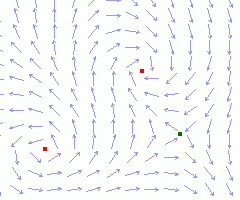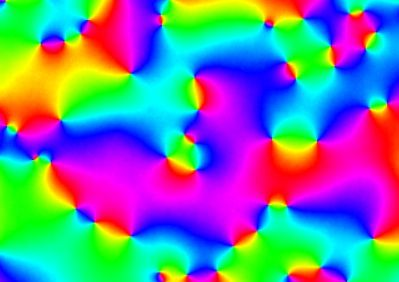it T mag   fps
Monte-Carlo simulations of spins dynamics in the XY magnetic model. Similar to the Ising model spins with different orientation φ are painted in different colors Hue(φ/2π). Vortices and anti-vortices correspond to the singular points (defects) where all colors meet together. For T = 0.03 "hot" (random) spins are frozen in correlated clusters. You can watch vortices and anti-vortices formation and annihilation. Set in the T field T = 0.003 to freez spins. 1024x1024 lattice.

# XY modelIn the XY model spins rotate in a plane (see the picture to the left). Interaction energy of nearest neighbours pair is
Eij = -(si sj ) = -cos(φi - φj ) ,
where i-th spin phase φi is measured e.g. from the horizontal axis in the counter-clockwise direction. Energy of spin interaction is minimal in ordered state, when all spins are aligned. Therefore on a 3D lattice at low temperatures there is a phase transition in ordered state with non-zero magnetization. However on a 2D lattice small fluctuations are accumulated on infinite lattice and destroy long order at any finite temperature.

In 2D XY model there are excitations with nontrivial topology - vortices and anti-vortices (marked by red and green squares). Under path-tracing around a vortex or anti-vortex spins complete revolution on ±2π. 2D XY model is dual to 2D Coulomb model. Vortices and anti-vortices correspond to electrical charges with different signs. Vortex and anti-vortex attract each other and annihilate.## Comments

Metropolis algorithm is used in this simulations. Interactin energies are calculated as
ΔEi = cos(φ + δφ - φi ) - cos(φ - φi ) = 2 sin(δφ) sin(φ + δφ/2 - φi ).
Contents     Previous: Potts model   Next: Exitations in 3D XY model
updated 11 Oct 2015